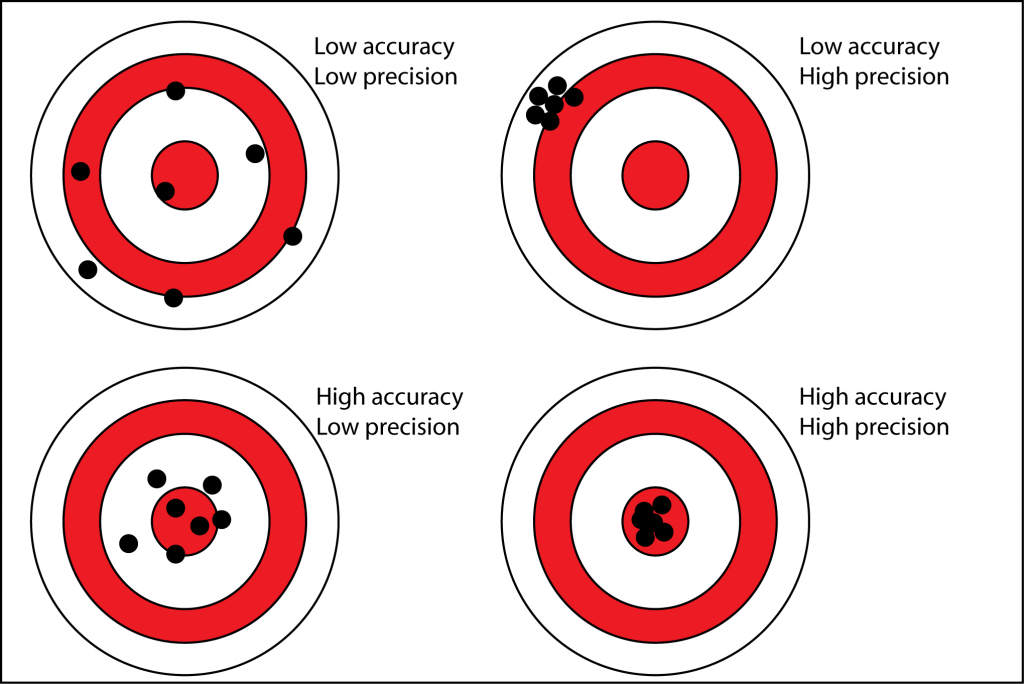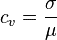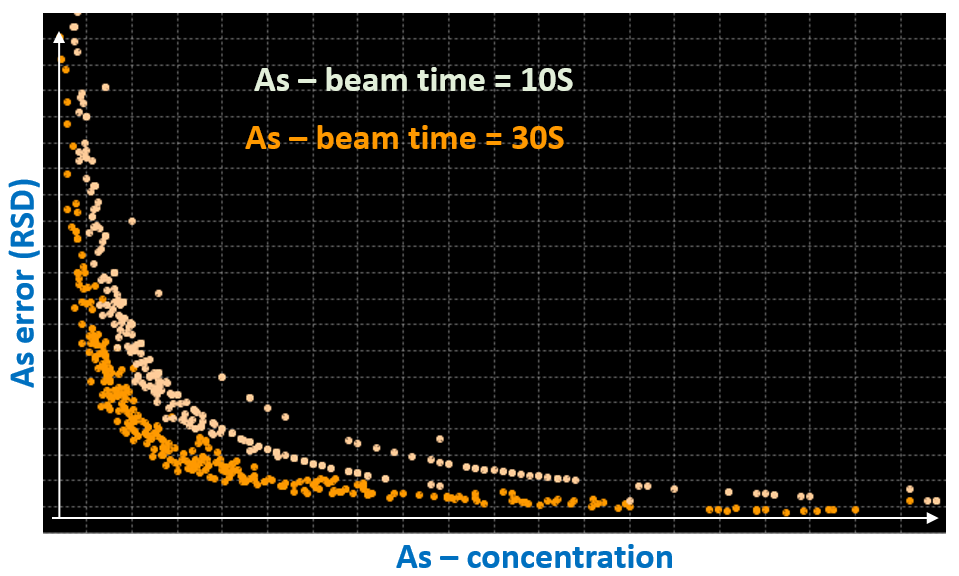^

# Precision and Accuracy

Precision and accuracy are two of the most important concepts to understand when thinking about pXRF technology.

### "pXRF instruments are precise but not accurate."

Automatically this statement can cause unease with pXRF users, but it shouldn’t. Understanding precision and accuracy will help any pXRF user embrace this statement!

## What is Precision?

Precision is a measure of variability or repeatability, or, how close your results are to each other. In terms of pXRF, precision is a measure of how well an instrument can measure something.

## What is Accuracy?

Accuracy is a measure of trueness or bias, how close the average value of your results is to the true value, or, how ‘right’ your value is. In analytical chemistry there is no such thing as a true value of course! But we use CRMs, as they are the next best thing.## Calculating Precision

To calculate precision you need to take multiple readings of the same thing. In real life, we might measure a standard or CRM 10 times for example.

Terms you will typically hear being used to describe precision in analytical chemistry are coefficient of variation (CV) and relative standard deviation (RSD). These are exactly the same thing and are a standardised way of measuring dispersion, the spread of your results.CV = standard deviation / mean

The lower the RSD, the smaller the spread of your results and the higher their precision.

Consider a real life example. Often we want to assess how well a pXRF instrument can measure an element, in this example, As. As the concentration of arsenic increases, the RSD, or error, decreases exponentially until there is a negligible noticeable reduction in error with increasing grade.

This example also demonstrates the difference in RSD with measurement time. Intuitively, longer measurement times provide more precise results but the user will often want to plot data like this in order to choose an ideal measurement time. Depending on your application, there’s probably not a lot of use in measuring a sample for an extra minute if it’s only going to give you a very slight increase in precision.

Plotting data this way also gives the user an indication of the true limit of detection (LOD) for the element in their sample matrix. This is likely to differ from the quoted manufacturers LOD.## Calculating Accuracy

This is the easy one, kind of! Accuracy is simply the difference between your measured and expected result. We can express accuracy in absolute or relative (percentage) terms.

Relative difference = (observed mean – certified value/certified value) x100

In terms of the work we do with pXRF this is usually as far as we need to go. But! As mentioned above, there is no true value in analytical chemistry. We are using a certified value from a CRM and we know that there is still some error within this. To really understand the accuracy of our measurement we also need to understand the errors in the certified value e.g. analytical error, preparation error, weighing error etc.

“pXRF instruments are precise but not accurate.”

We can now appreciate that pXRF instruments can precisely measure elements over certain grade ranges. More importantly, we understand how to measure the precision and calculate how the instruments precision varies under different conditions.

So, if we are happy that the instrument is precise for what we need it for, does it matter that it isn’t accurate? No. As long as we have known values to compare our precise data to it is very easy to simply shift our data to match our certified value. This is as simple as multiplying all your data by a factor.

This is one of the reasons why we use CRM’s with pXRF and the main reason why we check a certain number of our samples with a laboratory, so we can confirm that the relationship we expect to see with the assayed values and our pXRF remains constant.

Portable Spectral Services can help you with choosing and supplying the right CRM!

## Now Available

Portable Spectral Services’ Schedule of Hire, Support Services & Fees is now available!